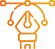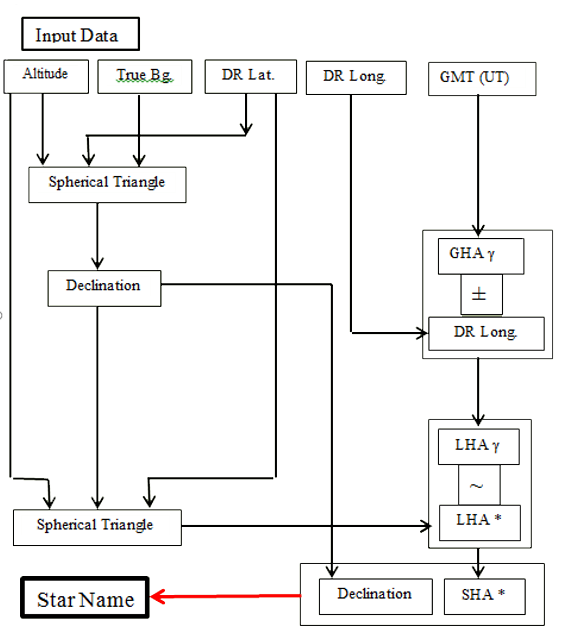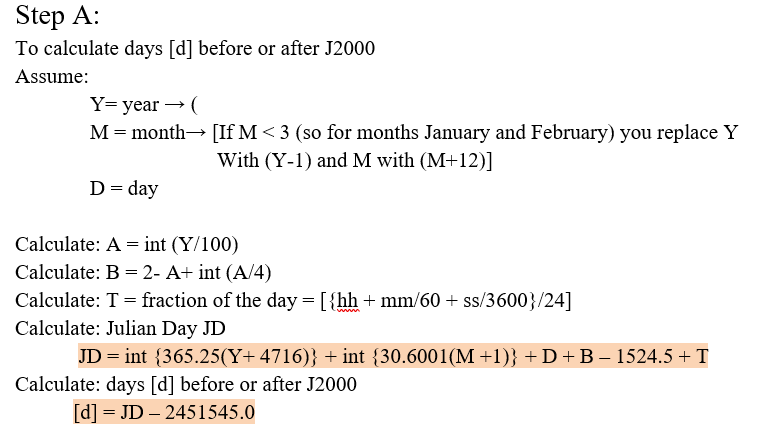### Identification of Unknown Star

Sometimes, after sailing for several days in overcast weather, a bright star appears through a gap between the clouds Because of the importance of getting the compass error constantly, it is necessary to seize this opportunity to get the compass error The first step, of course, is to determine the name of this star after observing its direction and altitude The current program is designed to solve this problem.

Apply it now### Coordinates of Sun and Aries and Equation of Time

This program was mainly designed to obtain the value of the equation of time (the link between the apparent solar time and the mean solar time) with the aim of using it in some of the previously mentioned programs such as the program for finding the times of the five daily prayers for example..

It is worth noting that while calculating the equation of time (Eq. of time), the coordinates of the sun are obtained, such as the declination (Dec.) and the Right Ascension (RA). Therefore, the program was developed to obtain the Greenwich Hour Angle for each of the Sun (GHA sun) and the Vernal Equinox Point. (GHA γ).

This is explained in the following steps:

Apply it now